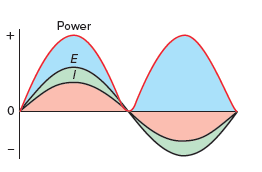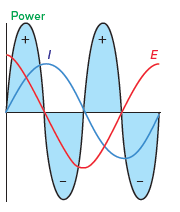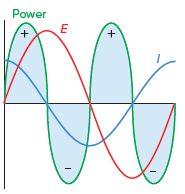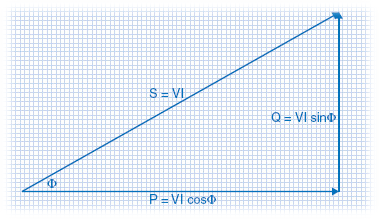Technical Article

# Understanding the Differences Between True Power, Reactive Power, and Apparent Power

July 28, 2021 by Alex Roderick

## True Power

True power is the power, measured in watts or kilowatts, used by motors, lights, and other devices to produce useful work or heat energy. True power is the resistive part of the circuit that performs the work. True power can be produced only when current and voltage are both positive or both negative (see Figure 1). If the current and voltage are out of phase, some of the currents do not produce useful work. Pure resistive circuits exist only in theory. In real situations, purely resistive circuits do not exist because the circuit conductors themselves produce some amount of inductance and capacitance.##### Figure 1. True power is the power, in watts or kilowatts, used by motors, lights, and other devices to produce useful work or heat energy.

Many loads are mainly resistive and make only very small inductive and capacitive contributions. These loads draw true power and are rated in watts (W). Therefore, it is appropriate to label primarily resistive loads in watts or kilowatts and ignore the reactive component. Examples of resistive loads are incandescent light bulbs, water heaters, unit heaters, hairdryers, and cooking ranges. Resistive loads have very small reactive components. Therefore, an apparent power rating (VA) would not be appropriate for these loads.

## Reactive Power

Reactive power is the power, measured in VAR or kVAR, released and stored by capacitors and inductors. It is the power that flows back into the source from the inductors and capacitors. It is this opposing power that affects the power factor of a circuit.

In a circuit with reactive components, the voltage and current are out of phase. For inductive circuits, the current lags the voltage (see Figure 2). Power is being absorbed by the circuit at those times when the voltage and current are in the same direction (both positive or both negative). Power is returned to the source when the voltage and current are not in the same direction (one positive and one negative).##### Figure 2. Reactive power is the power, in VAR or kVAR, stored and released by inductors and capacitors.

Reactive power is returned to the source without being consumed. However, current flows through the circuit to supply the reactive power. Wires, components, and devices must be sized to allow for the increased current flow from the reactive power.

## Apparent Power

Apparent power is power, in VA or kVA, that is the vector sum of true power and reactive power. Apparent power is the product of the total current and voltage in a circuit. Many loads include reactive components. Apparent power, in VA, is made up of the true power consumed by resistive loads and the reactive power flowing through capacitive and inductive loads. A common type of inductive load is an inductive motor. Inductive loads have the current lagging the voltage. In addition, many circuits contain harmonics. Harmonics contribute to apparent power.##### Figure 3. Apparent power is a vector sum of Real and Reactive Power

The following expression is used to compute the apparent power:

$$S=\sqrt{{{P}^{2}}+{{Q}^{2}}}$$

where S, P, and Q denote apparent power, true power, and reactive power, respectively.

## Power Factor

Power factor (PF) is the ratio of true power used in an AC circuit to apparent power delivered to the circuit. Power factor is expressed as a percentage. It is ideal for designing a system with a 95% power factor. An increase in reactive power (VAR) causes the power factor to decrease. The decreased power factor means more wasted power is used to perform the work. A utility company often penalizes customers for poor power factors because of the wasted power.

To calculate the power factor, apply the formula:

$$PF=\frac{{{P}_{T}}}{{{P}_{A}}}\times 100$$

PT = true power (in W)

PA = apparent power (in VA)

100 = constant (to convert decimal to percent)

A transformer operating under no-load conditions has a low power factor because the circuit is almost purely reactive. As the load on a transformer increases, the reactance decreases, and the power factor increases. At full load, the power factor approaches 1.

## Transformer Ratings

Transformers are rated in kVA because apparent power represents the total power (current × voltage) the transformer can supply. The single-phase total full-load current is calculated by dividing the power by the voltage. For example, a 25kVA transformer can deliver 104A to a 1φ load at 240V (25,000 ÷ 240 = 104A). The technician must ensure that the load connected to the transformer does not exceed the full current rating of the transformer.

$\begin{matrix}S=VI\text{ } & \text{For Single Phase} \\\end{matrix}$

$\begin{matrix}S=\sqrt{3}VI & \text{For Three Phase} \\\end{matrix}$

The actual power consumed by the load is in watts (W) or kilo-watts (kW). The power factor of a circuit can be calculated by dividing the kW consumed by the load by the kVA supplied by the transformer.

$Power\text{ }Factor=\frac{P(W)}{S(VA)}=\frac{P(kW)}{S(kVA)}$